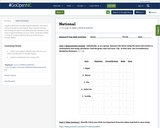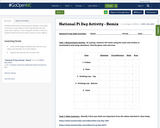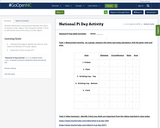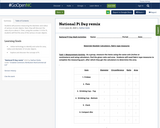Updating search results...

# 5 Results

View
Selected filters:
• NC.EX.Math.7.G.AConditional Remix & Share Permitted
CC BY-NC
Rating
0.0 stars

Students will practice measuring the diameter and radius of food themed circular objects. Next, they will discover that finding the ratio of circumference and diameter is the value of approximately 3.14 or pi.
Then, using the number 3.14 for Pi, students will find the area of the various circular objects.

Subject:
Algebra
Geometry
Math 1
Mathematics
Material Type:
Activity/Lab
Homework/Assignment
07/10/2020Conditional Remix & Share Permitted
CC BY-NC
Rating
0.0 stars

Students will practice measuring the diameter and radius of various circular objects. Next, they will discover the ratio of pi to about 3.
Then, using the number 3.14 for Pi, students will find the area of the various circular objects.

Subject:
Algebra
Geometry
Math 1
Mathematics
Material Type:
Activity/Lab
Homework/Assignment
11/06/2019Conditional Remix & Share Permitted
CC BY-NC
Rating
0.0 stars
Rating
0.0 stars
Subject:
Geometry
Mathematics
Material Type:
Lesson Plan
Author:
Nathan Rutko
07/31/2019Conditional Remix & Share Permitted
CC BY-NC
Rating
0.0 stars

Students will practice measuring the diameter and radius of various circular objects. Then using the number 3.14 for Pi, will find the area of the various circular objects.

Subject:
Algebra
Geometry
Math 1
Mathematics
Material Type:
Activity/Lab
Homework/Assignment
11/06/2019Conditional Remix & Share Permitted
CC BY-NC
Rating
0.0 stars

Students will practice measuring the diameter and radius of various circular objects. Next, they will discover the ratio of pi to about 3.
Then, using the number 3.14 for Pi, students will find the area of the various circular objects.

Subject:
Algebra
Geometry
Math 1
Mathematics
Material Type:
Activity/Lab
Homework/Assignment